# DK Goel Solutions Class 11 Accountancy Chapter 6 Accounting Equations

Read DK Goel Class 11 Accountancy Solutions for Chapter 6 Accounting Equations below. These DK Goel Accountancy Class 11 solutions have been prepared based on the latest book for DK Goel Class 11 for the current academic year by expert accounts teachers at studiestoday.com. These DK Goel Class 11 Solutions help commerce students in class 11 understand accountancy and build a strong base in accounts. Students in Class 11 who study accountancy and use the DK Goel Accountancy book to understand concepts of Chapter 6 Accounting Equations should understand the concepts and solve practice questions and exercises given at the end of the chapter. We have provided solutions for all questions and have also provided short notes for each problem. This will help Class 11 DK Goel Accountancy students to understand the questions properly. Refer to the solutions provided below prepared by CBSE NCERT teachers

## Chapter 6 Accounting Equations DK Goel Class 11 Solutions

Class 11 Accountancy students should read the following DK Goel Solutions for Class 11 Chapter 6 Accounting Equations in Standard 11. All solutions provided below can be downloaded in Pdf and are available for free. This DK Goel Book for Grade 11 Accountancy will be very useful for exams and help you to score good marks in Class 11 accountancy examinations. On our website www.studiestoday.com, we have provided solutions for all chapters given in the DK Goel Accountancy Book for Class 11.

### DK Goel Solutions Chapter 6 Accounting Equations Class 11 Accountancy

Question 1. Give two basic purposes of the accounting equation.

Solution 1: The two basic purposes of the accounting equation are.

(i) The accounting equation is always equal from both sides debit and credit. It shows the accuracy of recording of a financial transaction.

(ii) It helps in preparation of the final accounts.

Question 2. Which of the following equations are correct?

I. Assets = Capital + Liabilities

II. Assets = Capital – Liabilities

III. Assets = Liabilities – Capital

IV. Capital = Assets – Liabilities

V. Capital = Assets + Liabilities

VI. Liabilities = Capital + Assets

VII. Liabilities = Capital – Assets

VIII. Liabilities = Assets – Capital

Solution 2:  Below are the correct equations:-

I. Assets = Capital + Liabilities

IV. Capital = Assets – Liabilities

VIII. Liabilities = Assets – Capital

Question 3. The position of a businessman on 30th June 1994 was as follows –

Cash Rs. 5,000, Debtor Rs. 20,000, Machinery Rs. 60,000, Stock Rs. 25,000, Capital Rs. 75,000. Calculate his liabilities.

Solution 3:

Total Assets = Cash + Debtors + Machinery + Stock

= Rs. 5,000 + Rs. 20,000 + Rs. 60,000 + Rs. 25,000

= Rs. 1,10,000

Capital = Rs. 75,000

Liabilities = Assets - Capital

Liabilities = Rs. 1,10,000 – Rs. 75,000

= Rs. 35,000

Question 4. What entry (debit or credit) would you make to

(a) Increase in revenue

(b) Decrease in expense

(c) Record drawing

(d) Record the fresh capital introduced by owner

Solution 4:

(a)     Increase in revenue                                                            Credit

(b)    Decrease in expense                                                           Credit

(c)     Record drawing                                                         Debit in Capital Account

(d)    Record the fresh capital introduced by owner              Credit in Capital Account

Question 5. If a transaction has the effect of decreasing an asset, is the decrease recorded as a debit or credit? If the transaction has the effect of decreasing a liability, is the decrease recorded as a debit or credit?

Solution 5:

(i) A decrease in Asset will be the credit.

(ii) A Decrease in Liability will be the debit.

Question 6.

Name the transaction that will

(i) Decrease the assets and decrease the capital

(ii) Increase the assets and increase the liabilities

(iii) Increase the assets and decrease another asset

(iv) Decrease the assets and decrease the liabilities

Solution 6:

(i) Decrease the assets and decrease the capital – Cash withdraw for personal use Drawings.

(ii) Increase the assets and increase the liabilities – Purchase an asset on credit basis.

(iii) Increase the assets and decrease another asset – Sale or purchases of stock on cash basis.

(iv) Decrease the assets and decrease the liabilities – Paid amount to creditors.

Question 7. What will be the effect of the following on the accounting equation-

(i) Purchased goods for Rs. 20,000 form Mahesh on credit

(ii) Sold goods to Suresh costing Rs. 8,000 for Rs. 10,000 in cash

(iii) Paid wages Rs. 500

(iv) Withdrew in cash for private use Rs. 2,000

(v) Paid to creditors Rs. 2,000

Solution 7:

(i) Increases Stock by Rs. 20,000 on assets side and Increases Creditors by Rs. 20,000 on liabilities side.

(ii) Increase Cash by Rs. 10,000 on assets side, decrease Stock by Rs. 8,000 on assets side and increase Capital by Rs. 2,000.

(iii) Decrease Cash by Rs. 500 on assets side and decrease Capital by Rs. 500.

(iv) Decrease Cash by Rs. 2,000 on assets side and decrease Capital by Rs. 2,000.

(v) Decrease Cash by Rs. 2,000 Cash  and decrease creditor by Rs. 2,000 on liabilities side.

Question 8. If the total asset of a business is Rs. 2,00,000 and the net worth (capital) is Rs. 1,50,000. Calculate creditors.

Solution 8:

Liabilities = Assets – Capital (Net worth)

Creditors = Rs. 2,00,000 - Rs. 1,50,000                          (We know that creditors are liability.)

Creditors = Rs. 50,000

Question 9. A business on 1st April 2011 with a capital of Rs. 5,00,000. On 31st March 2012, his assets were worth Rs. 7,80,000 and liabilities Rs. 70,000. Find out his closing capital and profits earned during the year.

Solution 9:

Closing Capital = Closing Assets - Closing liabilities

= Rs. 7,80,000 – Rs. 70,000

= Rs. 7,10,000

Profit = Closing Capital – Opening Capital

= Rs. 7,10,000 - Rs.  5,00,000

= Rs. 2,10,000

Question 10. On which side will the increase in the following account be recorded? Also, mention the nature of the account.

1. Cash

2. Machinery

3. Debtor

4. Creditor

5. Proprietor’s Account

7. Salary Paid

Solution 10:Question 11. On which side will the increase in the following account be recorded? Also, mention the nature of the account.

1. Furniture

2. Bank

3. Proprietor’s Account

4. Salary Paid

5.Salary Outstanding

6. Subash - A Customer

Solution 11: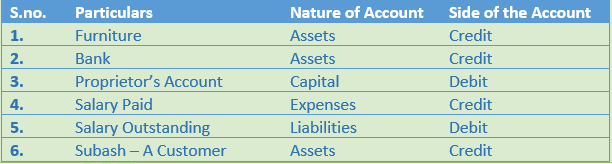Very Short Question

Question 1. What is an accounting equation?

Solution 1: An Accounting Equation is a mathematical expression which shows that the assets and liabilities of a firm are equal. An Accounting Equation is based on the dual aspect concept of accounting meaning; every transaction has two aspects- debit and credit. It holds that for every debit there is a credit of equal amount and vice versa.

Question 2. Give fundamental accounting equation.

Solution  2: The fundamental accounting equation is:-

Assets = Liabilities + Capital

Question 3. If the capital of a firm is Rs. 5,00,000 and outside liabilities are Rs. 2,00,000. Evaluate total assets of the company.

Solution  3: Capital of the firm = Rs. 5,00,000

Liabilities = Rs. 2,00,000

Assets = Liabilities + Capital

= Rs. 5,00,000 + Rs. 2,00,000

= Rs. 7,00,000

Hence, the Total Assets will be Rs. 7,00,000

Question 4. If total assets of a company are Rs. 10,00,000 and capital is Rs. 4,00,000. Calculate creditors.

Solution  4: Liabilities = Assets – Capital (Net worth)

Creditors = Rs. 10,00,000 - Rs. 4,00,000 (We know that creditors are liability)

Creditors = Rs. 6,00,000

Question 5. ‘X’ commenced business on April 1st 2013 with a capital of Rs. 6,00,000. On 31st March, 2014 his assets were worth Rs. 8,00,000 and liabilities Rs. 50,000. Find out his closing capital and profits earned during the year.

Solution 5: Closing Capital = Closing Assets - Closing liabilities

= Rs. 8,00,000 – Rs. 50,000

= Rs. 7,50,000

Profit = Closing Capital – Opening Capital

= Rs. 7,50,000 - Rs. 6,00,000

= Rs. 1,50,000

Question 6. What is debit?

Solution  6: Debit is the output of increase in assets and decrease in liabilities. Debits are the balance of credits these are the opposing each other. When an amount is entered on the left-hand side of an account it is called debit.

Question 7. What is credit?

Solution  7: Credit is the output of decrease in assets and increase in liabilities. Credits are the balance of debits these are the opposing each other. When an amount is entered on the right-hand side of an account it is called credit.

Question 8. Why are the rules of credit and debit same for both capital and liabilities?

Solution  8: The rules of credit and debit same for both capital and liabilities because as per business entity concept the owner and business are the different entities. It is assumes that the owner of the business are creditors to the business firm and business firm is label to pay their owners.

Question 9. Name the side on which increase in capital is recorded.

Solution  9: Increase in the capital is recoded on the Credit side.

Numerical Questions:-

Question 1. Prepare Accounting Equation from the following:-

Amount(Rs.)

1.     Sandeep started business with Cash                                            1,00,000

2.     Purchases furniture for cash                                                              5,000

3.     Purchases goods for cash                                                                 20,000

4.     Purchases goods on credit                                                               36,000

5.     Paid for rent                                                                                           700

6.     Goods costing Rs. 40,000 sold at a price of 20% for cash

Solution 1:Working Note:-

(i) In transaction 6 goods costing Rs. 40,000 sold on 20% profit (40,000 × 20%) = 40,000 × = Rs. 8,000.

Selling price of goods = 40,000 + 8,000 = Rs. 48,000.

(ii) Total Assets = Cash + Furniture + Stock

= 1,22,300 + 5,000 +16,000

= 1,43,300

Liabilities = Creditors

= 36,000

Capital = Assets – Liabilities

= 1,43,300 – 36,000

= 1,07,000

Point of Knowledge:-

Accounting equation is based on the dual concept of accounting, according to which, every transaction has two aspects namely Debit and Credit. It means that every transaction in accounting affects both Debit (Dr.) and Credit (Cr.)

Question 2. (A) Show the Accounting Equation on the basis of the following and present a balance sheet on the last new equation balances:

Amount  (Rs.)

1. Manu started business with cash                                       50,000
2. Bought furniture for                                                              500
3. Purchased goods on credit                                                  4,000
4. Sold goods on cash (cost Rs. 500) for                                    700
6. Purchased goods for cash                                                   1,000
7. Withdrew for personal use                                                     700
8. Paid to creditors                                                                   400
9. Paid for salaries                                                                    200

Solution 2: (A)Balance Sheet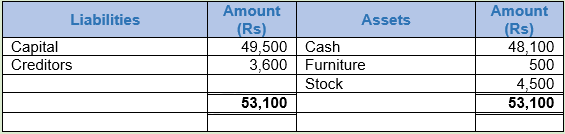Working Note:-

(i) In transaction 4 goods costing Rs. 500 sold at Rs. 700. So, the profit will be Rs. 700 - Rs. 500 = Rs. 200 it will rise our capital by Rs. 200.

(ii) Total Assets = Cash + Furniture + Stock

= 48,100 + 500 +4,500

= 53,100

Liabilities = Creditors

= 3,600

Capital = Assets – Liabilities

= 53,100 – 3,600

= 49,500

Point of Knowledge:-

Accounting equation thus refers to an equation in which total assets are always equal to the total Liabilities (i.e. Capital + Liabilities).

Question 2. (B) Prove that the accounting equation is satisfied in all the following transactions of Rajaram. Also prepare a balance sheet:-

1. Started business with cash Rs. 1,20,000
2. Purchased a typewriter for cash Rs. 8,000 for office use
3. Purchased goods for Rs. 50,000 on cash
4. Purchased goods for Rs. 40,000 on credit
5. Goods costing Rs. 60,00 sold for Rs. 80,000 on credit
6. Paid for rent Rs. 1,500 and for salaries Rs. 2,000
7. Received Rs. 800 for commission
8. Withdrew for private use Rs. 5,000 in cash

Solution 2 (B):Balance SheetWorking Note:-

(i) In transaction 5 goods costing Rs. 60,000 sold at Rs. 80,000. So, the profit will be Rs. 80,000 - Rs. 60,000 = Rs. 20,000 it will rise our capital by Rs. 20,000.

(ii) Total Assets = Cash +Typewriter + Stock + Debtors

= 54,300 + 8,000 + 30,000 + 80,000

= 1,72,300

Liabilities = Creditors

= 40,000

Capital = Assets – Liabilities

= 1,72,300 – 40,000

= 1,32,300

Point of Knowledge:-

Accounting equation thus refers to an equation in which total assets are always equal to the total Liabilities (i.e. Capital + Liabilities).

Question 3. Prepare Accounting Equation from the following:

(a) Started business with Cash Rs. 2,00,000.

(b) Purchased goods for Cash Rs. 60,000 and on Credit Rs. 1,50,000.

(c) Sold goods for Cash costing Rs. 40,000 at a profit of 20% and on Credit costing Rs. 72,000 at a profit of 25%.

(d) Paid for Rent Rs. 5,000.

Solution 3: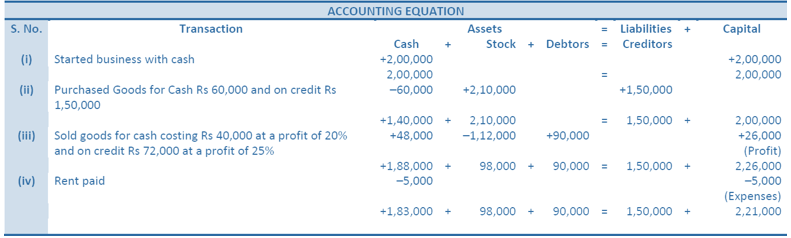Working Note:-

(i) In transaction 3 the

Cost of goods sold is Rs. 40,000. Sold on cash 20% of profit Rs. 40,000 ×20/100 = Rs. 8,000.

Selling price = Rs. 40,000 + Rs. 8,000 = Rs. 48,000.

Cost of goods sold is Rs. 72,000. Sold on cash 20% of profit Rs. 72,000 ×25/100 = Rs. 18,000.

Selling price = Rs. 72,000 + Rs. 18,000 = Rs. 90,000.

Total cost of goods sold = Rs. 40,000 + 72,000 = 1,12,000.

(ii) Total Assets = Cash + Stock + Debtors

= 1,83,000 + 98,000 + 90,000

= 3,71,000

Liabilities = Creditors

= 1,50,000

Capital = Assets – Liabilities

= 3,71,000 – 1,50,000

= 2,21,000

Point of Knowledge:-

The accounting equation is always equal from both sides debit and credit. It shows the accuracy of recording of a financial transaction.

Question 4. Prepare accounting equation from the following.

Amount(Rs.)

1. Kunal started business with cash                       2,50,000
2. He purchased furniture for cash                            35,000
3. He paid commission                                                 2,000
4. He purchased goods on credit                               40,000
5. He sold goods(Costing Rs.20,000) for cash          26,000

Solution 4:Working Note:-

(i) In transaction 4 the cost of goods Rs. 20,000 sold at Rs. 26,000. So, the profit will be Rs. 26,000 - Rs. 20,000 = Rs. 6,000.

(ii) Total Assets = Cash + Furniture + Stock

= 2,39,000 + 35,000 + 20,000

= 2,94,000

Liabilities = Creditors

= 40,000

Capital = Assets – Liabilities

= 2,94,000 – 40,000

= 2,54,000

Point of Knowledge:-

The accounting equation is always equal from both sides debit and credit. It shows the accuracy of recording of a financial transaction.

Question 5. Mohit has the following transaction. Prepare accounting equation.

Amount (Rs.)

1. Business started with cash                                                       1,75,000
2. Purchased goods from Rohit                                                        50,000
3. Sold goods on credit to Manish (costing Rs.17,500)                  20,000
4. Purchased furniture for office use                                               10,000
5. Cash paid to Rohit in full settlement                                            48,500
6. Cash received from Manish                                                           20,000
7. Rent Paid                                                                                          1,000
8. Cash withdrawn for personal use                                                   3,000

Solution 5: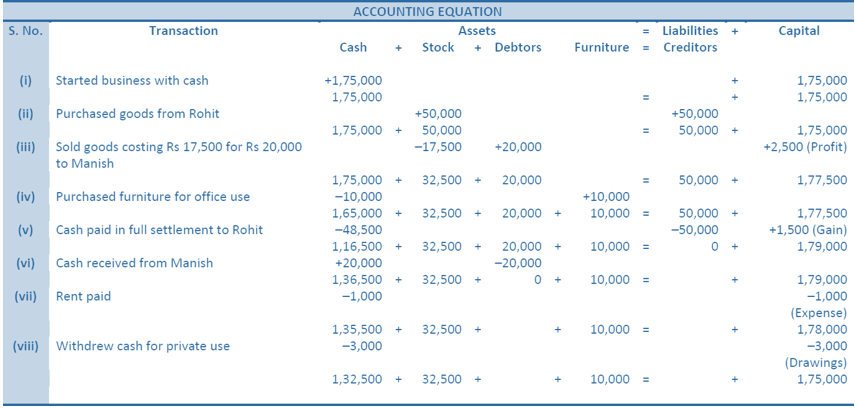Working Note:-

(i) In transaction 3 the cost of goods Rs. 17,500 sold at Rs. 20,000. So, the profit will be Rs. 17,500 - Rs. 20,000 = Rs. 2,500.

(ii) Total Assets = Cash + Stock + Debtors + Furniture

= 1,32,500 + 32,500 + 0 + 10,000

= 1,75,000

Liabilities = Creditors

= 0

Capital = Assets – Liabilities

= 1,75,000 – 0

= 1,75,000

Point of Knowledge:-

Every transaction has two aspects- debit and credit. It holds that for every debit there is a credit of equal amount and vice versa.

Question 6. What will be the effect of the following on the Accounting Equation?

(i) Harish started business with cash Rs. 1,80,000

(ii) Purchased goods for cash Rs. 60,000 and on credit Rs. 30,000

(iii) Sold goods for cash Rs. 40,000; costing Rs. 24,000

(iv) Rent paid Rs. 5,000; and rent outstanding Rs. 2,000

(v) Sold goods on credit Rs. 50,000 (costing Rs. 38,000)

(vi) Salary paid in advance Rs. 3,000

Solution 6: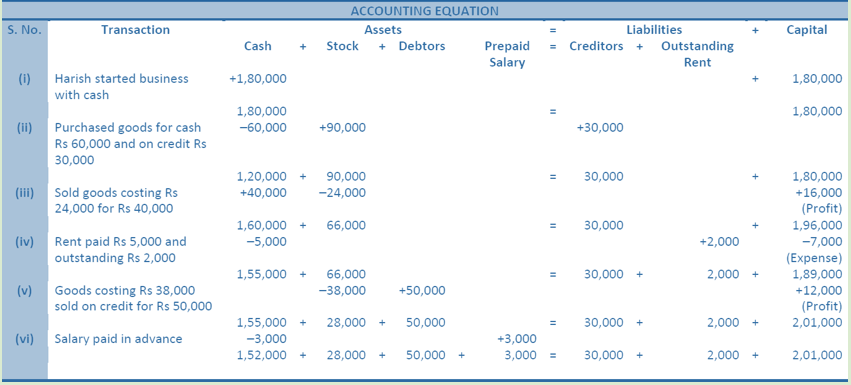Working Note:-

(i) In transaction 3 the cost of goods Rs. 24,000 sold at Rs. 40,000. So, the profit will be Rs. 17,500 - Rs. 20,000 = Rs. 16,000.

(ii) Total Assets = Cash + Stock + Debtors + Prepaid Salary

= 1,52,000 + 28,000 + 50,000 + 3,000

= 2,33,000

Liabilities = Creditors + Outstanding Rent

= 30,000 + 2,000

= 32,000

Capital = Assets – Liabilities

= 2,33,000 – 32,000

= 2,01,000

Point of Knowledge:-

Every transaction has two aspects- debit and credit. It holds that for every debit there is a credit of equal amount and vice versa.

Question 7. Use Accounting Equation to show the effect of the following transactions of M/s Royal Traders.

Amount (Rs.)

1. Business started with cash                                  1,20,000
2. Purchased goods for cash                                       10,000
4. Salary outstanding                                                     2,000
5. Prepaid insurance                                                      1,000
7. Sold goods for cash(costing Rs.5,000)                     7,000
8. Goods destroyed by fire                                                500

Solution 7:Working Note:-

(i) Total Assets = Cash + Stock + Prepaid Insurance

= 1,14,700 + 10,000 + 1,000 + 2,000

= 1,25,700

Liabilities = Outstanding Salary

= 2,000

= 2,000

Capital = Assets – Liabilities

= 1,25,700 – 2,000

= 1,23,700

Point of Knowledge:-

Outstanding transactions are the liability for the business firm and prepaid transaction are the assets for the business firm.

Question 8.

(A) Prepare Accounting Equation from the following.

1. Started business with cash Rs. 75,000 and goods Rs. 25,000
2. Paid for Rent Rs. 2,000
3. Bought goods for cash Rs. 30,000 and on credit for Rs. 44,000
4. Goods costing Rs. 50,000 sold at a profit of 25%, out of which Rs. 27,500 received in Cash
5. Purchased a Motor-cycle for personal use Rs. 20,000

Solution 8: (A)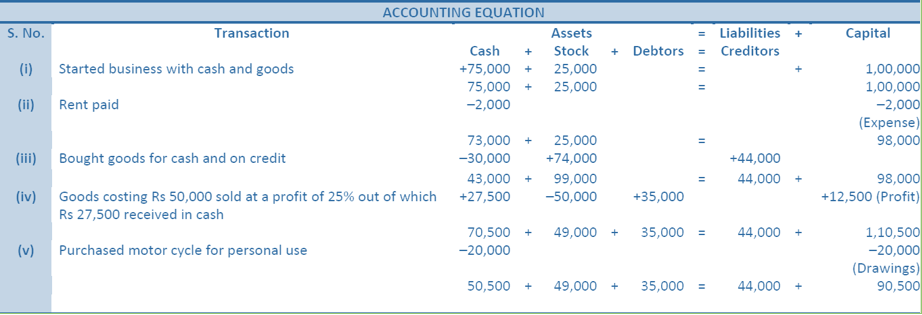Working Note:-

(i) In transaction 4 the cost of goods Rs. 50,000 sold at Rs. 25% of profit. So, the profit will be Rs. 50,000 × 25/100 = Rs. 12,500. Selling price = Rs. 50,000 + Rs. 12,500 = Rs. 62,500. In which Cash Sale = 27,500 and Credit Sale = Rs. 35,000.

(ii) Total Assets = Cash + Stock + Debtors

= 50,500 + 49,000 + 35,000

= 1,34,500

Liabilities = Creditors

= 44,000

= 44,000

Capital = Assets – Liabilities

= 1,34,500 – 44,000

= 90,500

Point of Knowledge:-

Every transaction has two aspects- debit and credit. It holds that for every debit there is a credit of equal amount and vice versa.

Question 8.

(B) Prepare Accounting Equation from the following and also prepare a Balance Sheet:-

1. Raghu started business with Cash Rs.1,50,000
2. Bought goods for cash Rs.80,000 and on credit for Rs.40,000
3. Goods costing Rs.75,000 sold at a profit of 33½%. Half the payment received in cash
4. Goods costing Rs.10,000 sold for Rs.12,000 on credit
5. Paid for Rent Rs.2,000 and for salaries Rs.4,000
6. Goods costing Rs.20,000 sold for Rs.18,500 for Cash

Solution 8: (B)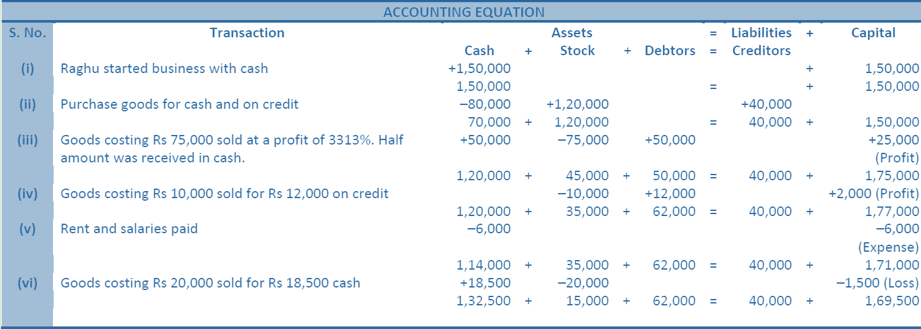Balance SheetWorking Note:-

(i) In transaction 3 the cost of goods Rs. 75,000 sold at Rs. 100/3% of profit. So, the profit will be Rs. 75,000 × 100/3% = Rs. 25,000. Selling price = Rs. 75,000 + Rs. 25,000 = Rs. 1,00,000. In which Cash Sale = 50,000 and Credit Sale = Rs. 50,000.

(ii) Total Assets = Cash + Stock + Debtors

= 1,32,500 + 15,000 + 62,000

= 2,09,500

Liabilities = Creditors

= 40,000

= 40,000

Capital = Assets – Liabilities

= 2,09,500 – 40,000

= 1,69,500

Point of Knowledge:-

Every transaction has two aspects- debit and credit. It holds that for every debit there is a credit of equal amount and vice versa.

Question 9. If the Capital of a business is Rs. 1,20,000 and Outside liabilities are Rs. 20,000, calculate total assets of the business.

Solution  9: We know that

Assets = Liabilities + Capital

Assets = Rs. 20,000 + Rs. 1,20,000

Assets = Rs. 1,40,000

Point of Knowledge:-

The formulas using to calculate Assets, Liabilities and Capital are:-

Assets = Liabilities + Capital

Liabilities = Assets – Capital

Capital = Assets – Liabilities

Question 10. If total assets of a business are Rs. 1,30,000 and capital is Rs. 80,000, calculate creditors.

Solution  10 :  Assets = Rs. 1,30,000

Capital = Rs. 80,000

We know that

Liabilities = Assets – Capital

Liabilities = Rs. 1,30,000 – Rs. 80,000

Liabilities = Rs. 50,000

Point of Knowledge:-

The accounting equation is always equal from both sides debit and credit. It shows the accuracy of recording of a financial transaction.

Question 11. ‘A’ commenced his cloth business on 1st April, 2011 with a capital of Rs. 3,00,000. On 31st March, 2012 his assets were worth Rs. 5,00,000 and liabilities Rs. 1,00,000. Find out his closing capital and profits earned during the year.

Solution  11: Opening Capital = Rs. 3,00,000

Assets = Rs. 5,00,000

Liabilities = Rs. 1,00,000

Calculation of Closing Capital

Closing Capital = Assets – Liabilities

Closing Capital = Rs. 5,00,000 – Rs. 1,00,000

Closing Capital = Rs. 4,00,000

Calculation of Profit

Profit = Closing Capital – Opening Capital

Profit = Rs. 4,00,000 – Rs. 3,00,000

Profit = Rs. 1,00,000

Point of Knowledge:-

Closing Capital = Opening Capital + Additional Capital + Profit - Drawings

Question 12. (A) Yogesh commenced business on 1st April, 2011 with a Capital of Rs. 5,00,000 and a loan of Rs. 1,00,000 borrowed from Citi Bank. On 31st March, 2012, his assets were Rs. 8,00,000. Calculate his closing capital and profits earned during the year.

Solution 12:

(A) Opening Capital = Rs. 5,00,000

Assets = Rs. 8,00,000

Liabilities (Loan) = Rs. 1,00,000

Calculation of Closing Capital

Closing Capital = Assets – Liabilities

Closing Capital = Rs. 8,00,000 – Rs. 1,00,000

Closing Capital = Rs. 7,00,000

Calculation of Profit

Profit = Closing Capital – Opening Capital

Profit = Rs. 7,00,000 – Rs. 5,00,000

Profit = Rs. 2,00,000

Working Capital:-

(i) It is assumed that loan borrowed from Citi Bank has not been paid till the end of the accounting year.

Point of Knowledge:-

(i) Closing Capital = Opening Capital + Additional Capital + Profit – Drawings

Question 12. (B) If in the above case, the proprietor had introduced fresh capital of Rs. 40,000 and had withdrawn Rs. 10,000 for personal purposes, calculate his profits.

Solution 12:

(B) Opening Capital = Rs. 5,00,000

Assets = Rs. 8,00,000

Liabilities (Loan) = Rs. 1,00,000

Calculation of Closing Capital

Closing Capital = Assets – Liabilities

Closing Capital = Rs. 8,00,000 – Rs. 1,00,000

Closing Capital = Rs. 7,00,000

Calculation of Profit

Closing Capital = Opening Capital + Additional Capital + Profit – Drawings

Rs. 7,00,000 = Rs. 5,00,000 + Rs. 40,000 + Profit – Rs. 10,000

Rs. 7,00,000 = Rs. 5,30,000 + Profit

Rs. 7,00,000 - Rs. 5,30,000 = Profit

Profit = Rs. 7,00,000 - Rs. 5,30,000

Profit = Rs. 1,70,000

Point of Knowledge:-

(i) Closing Capital = Opening Capital + Additional Capital + Profit – Drawings

Question 13. Give one example of each of the following transactions

1. Increase in an asset and a liability
2. Decrease in an asset and a liability
3. Increase in assets and capital
4. Decrease in assets and capital

Solution 13:

1.) Transaction - Increase in an asset and a liability.

Example :- Goods purchases on credit basis.

2.) Transaction - Decrease in an asset and a liability.

Example :- Amount paid to creditors.

3.) Transaction - Increase in assets and capital.

4.) Decrease in assets and capital.

Example :- Amount withdraw by proprietor.

Question 14. On which side the increase in the following accounts will be recorded? Also mention the nature of account:−

1. Furniture
2. Rent Paid
4. Salary Paid
5. Proprietor’s Paid
6. Debtor
7. Creditor

Solution 14:Question 15. On which side the decrease in the following accounts will be recorded? Also mention the nature of account:−

1. Cash Bank
2. Bank Overdraft
3. Rent Paid
4. Outstanding Rent
5. Prepaid Insurance
6. Manoj, Proprietor of the business

Solution 15: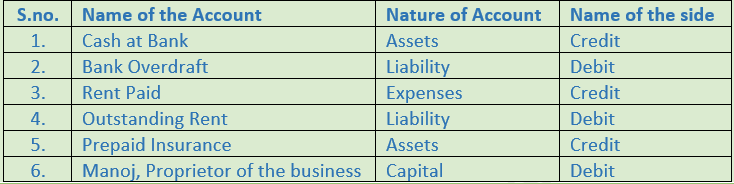Question 16. From the following transactions, state the nature of accounts and state the accounts which will be debited and credited:

1. Ganesh started business with Cash Rs. 2,00,000
2. Purchased goods for Cash Rs. 60,000
3. Sold goods for cash Rs. 75,000
4. Purchased goods from Nakul on Credit for Rs. 80,000
5. Sold goods to Bhushan on Credit for Rs. 50,000
6. Paid Cash to Nakul Rs. 20,000
7. Received Cash from Bhushan Rs. 10,000
8. Paid salary Rs. 20,000

Solution 16: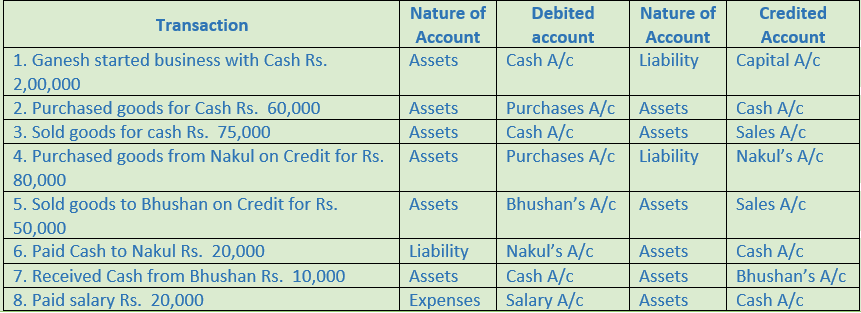Question 17. Open ‘T’ shape account for Machinery and write the following on the proper side.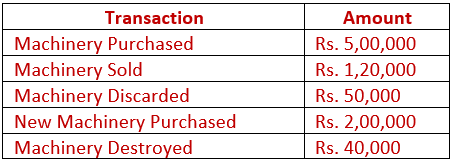Solution 17:                                                                                                    Machinery Account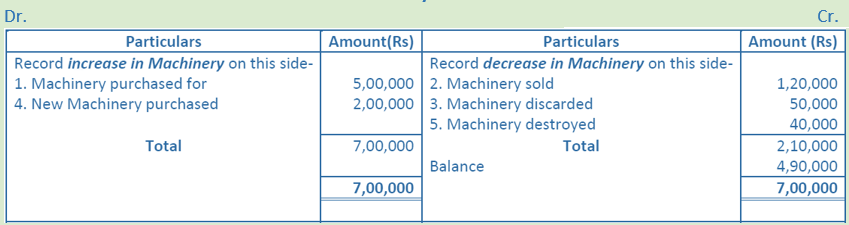Working Note:-

Increase in assets will be debit and decrease In assets will be credited. Here Machinery is an asset.

Question 18. Open ‘T’ shape account of our creditor ‘Raghubir’ and write the following transactions on the proper side.

1. Purchased goods from Raghubir on credit for Rs. 50,000
2. Returned goods to Raghubir for Rs. 5,000
3. Paid to Raghubir Rs. 30,000
4. Purchased goods from Raghubir on credit for Rs. 16,000
5. Paid to Raghubir Rs. 20,000

Solution 18:

Raghubir’s Account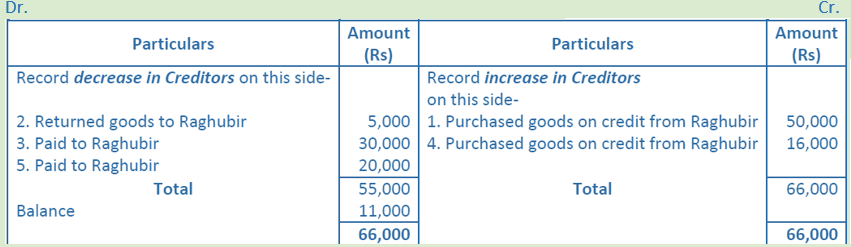Working Note:-

Decrease in liabilities will be debit and increase in liabilities will be credited. Here Raghubir is a creditor (liability).

Question 19. Put the following on the proper side of Cash account, Debtor’s account and Creditor’s account.

1. Sold goods for cash Rs. 60,000
2. Sold goods to Hari on credit Rs. 20,000
3. Purchased goods from Krishan on credit Rs. 36,000
4. Purchased goods from Krishan for cash Rs. 10,000
5. Cash received from Hari Rs. 15,000
6. Cash paid to Krishan Rs. 28,000

Solution 19:Point of Knowledge:-

1. Increase in asset will be debited and decrease will be credited.
2. Increase in the liabilities will be credited and decrease will be debited.
3. Increase in the capital will be credited and decrease will be debited.
4. Increase in the revenue or income will be credited and decrease will be debited.
5. Increase in expenses and losses will be debited and decrease will be credited.

Question 20. From the following transactions prepare the Proprietor’s Account in ‘T’ shape.Solution  20: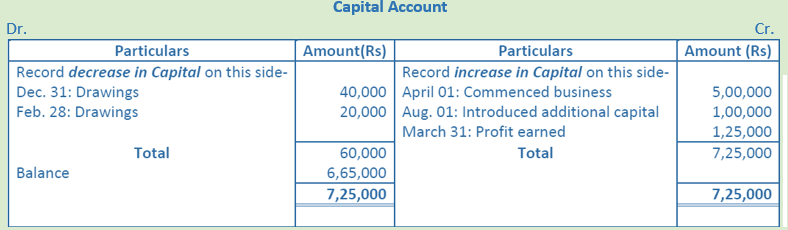Point of Knowledge:-

1. Increase in asset will be debited and decrease will be credited.
2. Increase in the liabilities will be credited and decrease will be debited.
3. Increase in the capital will be credited and decrease will be debited.
4. Increase in the revenue or income will be credited and decrease will be debited.
5. Increase in expenses and losses will be debited and decrease will be credited.

Question 21. Prepare the Accounting Equation on the basis of the following

1. Started business with cash Rs. 1,40,000 and Stock Rs. 2,50,000.
2. Sold goods (costing Rs. 50,000) at a profit of 25% on the cost.
3. Deposited into bank account Rs. 1,80,000.
4. Purchased goods from Mohan Rs. 80,000.

Solution 21:Working Note:-

(i) In transaction 2 goods costing Rs. 50,000 sold at 25% profit on cost = Rs. 50,000 ×25/100 = 12,500.

(ii) Total Assets = Cash + Stock + Bank

= 22,500 + 2,80,000 + 1,80,000

= 4,82,500

Liabilities = Creditors

= 80,000

Capital = Assets – Liabilities

= 4,82,500 – 80,000

= 4,02,500

Point of Knowledge:-

1. Increase in asset will be debited and decrease will be credited.
2. Increase in the liabilities will be credited and decrease will be debited.

Question 22. Prepare Accounting Equation on the basis of the following transactions.

1. Started business with cash Rs. 70,000.
2. Credit purchase of goods Rs. 18,000.
3. Payment made to creditors in full settlement Rs. 17,500.
4. Purchase of machinery for cash Rs. 20,000.

Solution 22:Working Note:-

(i) Amount paid to creditor Rs. 17,500 in full settlement instant of Rs. 18,000. Here the gap of Rs. 500 treated as discount received.

(ii) Total Assets = Cash + Stock + Machinery

= 32,500 + 18,000 + 20,000

= 70,500

Liabilities = Creditors

= 0

Capital = Assets – Liabilities

= 70,500 – 0

= 70,500

Point of Knowledge:-

The rules of credit and debit same for both capital and liabilities because as per business entity concept the owner and business are the different entities. It is assumes that the owner of the business are creditors to the business firm and business firm is label to pay their owners.

Question 23. Prepare accounting equation from the following

1. Started business with cash Rs. 50,000 and goods Rs. 30,000
2. Purchased goods for cash Rs. 30,000 and on credit from Karan Rs. 20,000
3. Goods costing Rs. 40,000 were sold for Rs. 55,000 for cash
4. Withdrew cash for personal use Rs. 10,000
5. Rent outstanding Rs. 2,000

Solution 23:Working Note:-

(i) In transaction 3 goods costing Rs. 40,000 sold at Rs. 55,000. Profit = Selling price – Cost Price

Profit = Rs. 55,000 – Rs. 40,000

Profit = Rs. 15,000

(ii) Total Assets      =     Cash + Stock

=     Rs. 65,000 + Rs. 40,000

=     Rs. 1,05,000

Liabilities         =     Creditors + Outstanding Rent

=     Rs. 20,000 + Rs. 2,000

=     Rs. 22,000

Capital              =     Assets – Liabilities

=     Rs. 1,05,000 – Rs. 22,000

=     Rs. 83,000

Point of Knowledge:-

In the accounting equation if the capital shows negative figure then it is drawings.

Question 24. Show the accounting equation on the basis of the following transactions and present a Balance Sheet of the last new equation balance.

Amount

Mohan commenced business with                                    Rs. 70,000

Purchased goods on Credit                                                Rs. 14,000

Withdrew for private use                                                   Rs. 1,700

Purchased goods for Cash                                                  Rs. 10,000

Paid wages                                                                         Rs. 300

Paid to Creditors                                                                Rs. 10,000

Sold goods on Credit at par                                                Rs. 15,000

Sold goods for Cash (cost price was Rs.  3,000)                Rs. 4,000

Purchased furniture for cash                                               Rs. 500

Solution 24: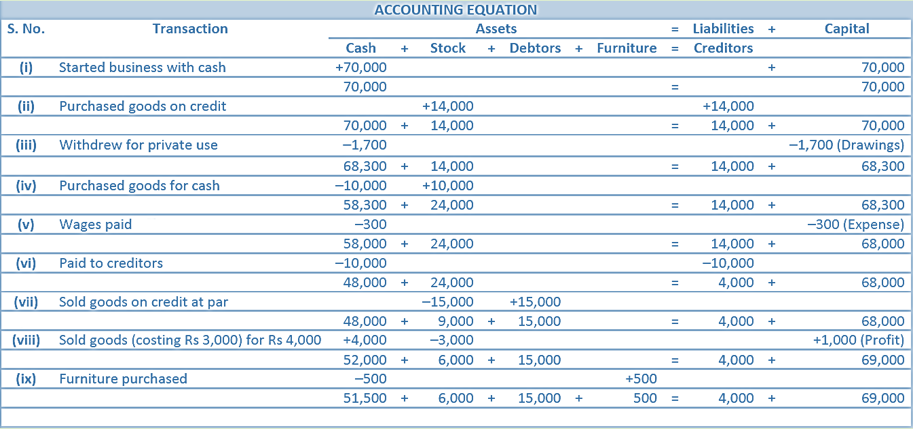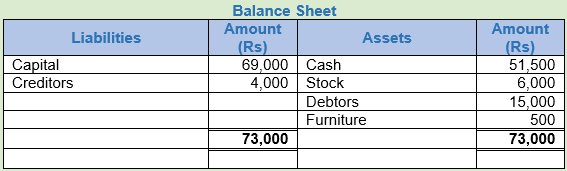Working Note:-

(i) In transaction 3 goods costing Rs. 3,000 sold at Rs. 4,000. Profit = Selling price – Cost Price

Profit = Rs. 4,000 – Rs. 3,000

Profit = Rs. 1,000

(ii) Total Assets = Cash + Stock + Debtors + Furniture

= Rs. 51,500 + Rs. 6,000 + Rs. 15,000 + Rs. 500

= Rs. 73,000

Liabilities = Creditors

= Rs. 4,000

Capital = Assets – Liabilities

= Rs. 73,000 – Rs. 4,000

= Rs. 69,000

Point of Knowledge:-

Accounting equation thus refers to an equation in which total assets are always equal to the total Liabilities (i.e. Capital + Liabilities).

Question 25. Prove that the accounting equation is satisfied in the following transactions.

Amount

Brij Mohan commenced business with Cash                                                                                       Rs. 1,00,000

Bought goods for Cash                                                                                                                         Rs. 60,000

1/3rd of the above goods sold at a profit of 20% on cost. Half the payment received in Cash

Purchased typewriter for office use                                                                                                    Rs. 15,000

Purchased goods on Credit from X                                                                                                      Rs. 25,000

Paid to X                                                                                                                                               Rs. 15,000

Paid Salary                                                                                                                                           Rs. 3,000

Sold goods for Cash (Cost Rs.  50,000)                                                                                              Rs. 60,000

Solution 25:Working Note:-

(i) In transaction 3 goods costing Rs. 50,000 sold at Rs. 60,000. Profit = Selling price – Cost Price

Profit = Rs. 60,000 – Rs. 50,000

Profit = Rs. 10,000

(ii) 1/3 part of total Goods = Rs. 60,000 ×1/3= Rs. 20,000

Rs. 60,000 × 1/3 = Rs. 20,000

Add: Profit (Rs. 20,000 ×20%) = Rs. 4,000

Selling price = Rs. 20,000 + Rs. 4,000 = Rs. 24,000

Cash Received = 24,000 × 50% = Rs. 12,000

Credit Sales = 24,000 × 50% = Rs. 12,000

(iii)

Total Assets = Cash + Stock + Typewriter + Debtors

= Rs. 79,500 + Rs. 15,000 + Rs. 15,000 + Rs. 12,000

= Rs. 1,21,500

Liabilities = Creditors

= Rs. 10,000

Capital = Assets – Liabilities

= Rs. 1,21,500 – Rs. 10,000

= Rs. 1,11,500

Point of Knowledge:-

Traditional or English rules of accounting:-

(1) Personal Account: Debit the receiver and credit the giver.

(2) Real Account: Debit what comes in and credit what goes out.

(3) Nominal Account: Debit all expenses and losses credit all incomes and gains.